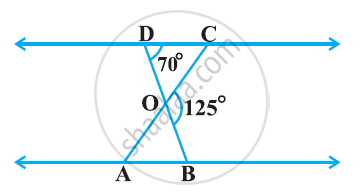# In the following figure, ΔODC ∼ ΔOBA, ∠BOC = 125° and ∠CDO = 70°. Find ∠DOC, ∠DCO and ∠OAB - Mathematics

In the following figure, ΔODC ∼ ΔOBA, ∠BOC = 125° and ∠CDO = 70°. Find ∠DOC, ∠DCO and ∠OAB#### Solution

DOB is a straight line.

∴ ∠DOC + ∠COB = 180°

⇒ ∠DOC = 180° − 125°

= 55°

In ΔDOC,

∠DCO + ∠CDO + ∠DOC = 180°

(Sum of the measures of the angles of a triangle is 180º.)

⇒ ∠DCO + 70º + 55º = 180°

⇒ ∠DCO = 55°

It is given that ΔODC ∼ ΔOBA.

∴ ∠OAB = ∠ OCD [Corresponding angles are equal in similar triangles.]

⇒ ∠OAB = 55°

Concept: Criteria for Similarity of Triangles
Is there an error in this question or solution?

#### APPEARS IN

NCERT Class 10 Maths
Chapter 6 Triangles
Exercise 6.3 | Q 2 | Page 139